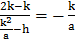# The locus of the mid-points of the portion of the normal to the parabolaintercepted between the curve and the axis is another parabola whose latus rectum is

## Question ID - 153535 :- The locus of the mid-points of the portion of the normal to the parabolaintercepted between the curve and the axis is another parabola whose latus rectum is

3537

(4)Consider the parabolaWe have to find the locus of, sincehas ordinate, ordinate ofisAlsois on the curve, then abscissa ofisNowis normal to curve

Slope of tangent to curve at any pointHence slope of normal at pointisAlso slope of normal joiningandisHence comparing slopesorForhence locus usNext Question :
 If the vertex of a hyperbola bisects the distance between its centre and the corresponding focus, then ratio of square of its conjugate axis to the square of its transverse axis is